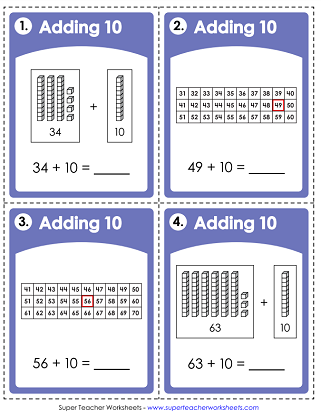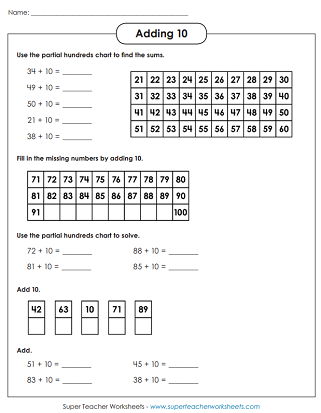# Mental Math: Adding 10 & Multiples of 10

Use models and/or mental math to add 10, or multiples of 10, to any 2-digit number. This page has printable worksheets, task cards, and flashcards.Use a hundreds chart to add 10 to each of the numbers shown.
Using mental math, add 10 to each 2-digit number. This worksheet has 8 horizontal problems and 2 word problems.
THese task cards have place value blocks and partial hundreds charts. Use the models or mental math to solve. 30 cards in all. Great for learning centers, classroom scavenger hunts, exit slips, or peer practice sessions.
Use mental math to add 10 more to each 2-digit number. This page has 26 vertical and horizontal problems.
Add 10 to the double-digit numbers. Then compare the sums.
example: 55 + 10 > 10 + 52

Use the partial hundreds chart to practice adding 2-digit numbers to multiples of 10.
(Example: 34+20=54)
This PDF contains 8 horizontal problems and 2 math stories (word problems).
This file has 30 illustrated task cards. Students use the pictures of place value blocks and hundreds charts to add multiples of 10.
(example: 49+30)
Add multiples of 10 to 2-digit numbers. There are 18 horizontal and 8 vertical problems.
(example: 54+30=84)
Compare addition problems with multiples of 10.
example: 34 + 30 < 43 + 20

STW has hundreds of printables for teaching all levels of basic and multi-digit addition.

## Sample Worksheet ImagesMy Account
Site Information